# 感知机模型

## 什么是感知机

$f(x)=sign(w^Tx+b)$,
\\sign(x)=\left\{\begin{aligned} 1 \qquad \quad x\ge0\\ -1 \qquad\quad x<0 \end{aligned}\right.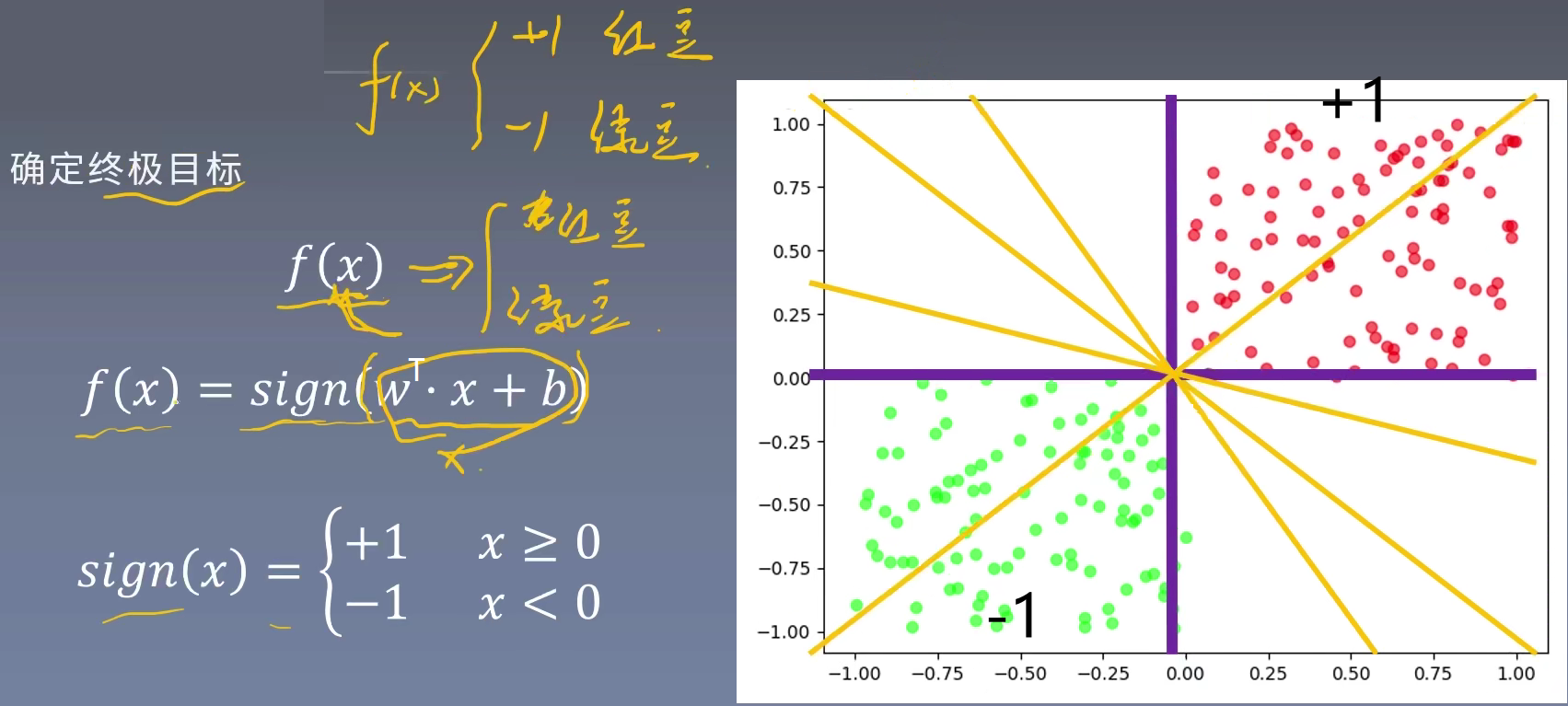# 感知机学习策略

## 什么是线性可分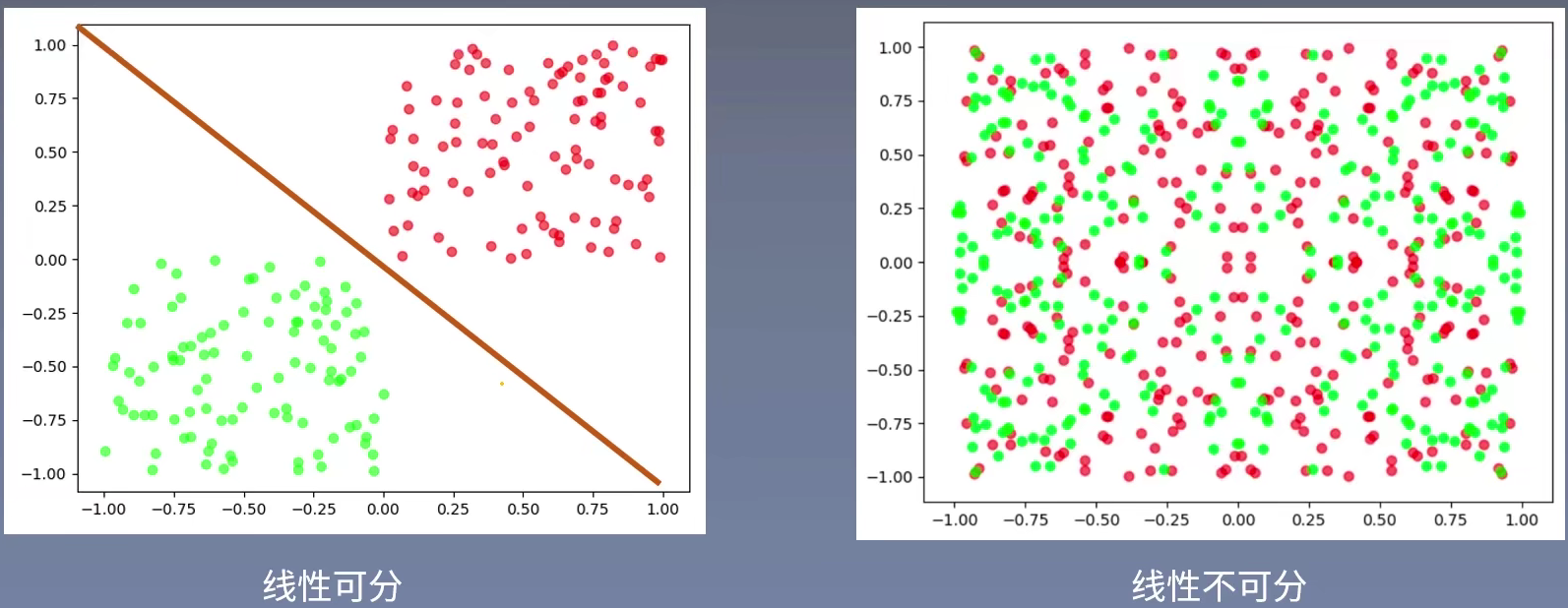## 几何间隔与函数间隔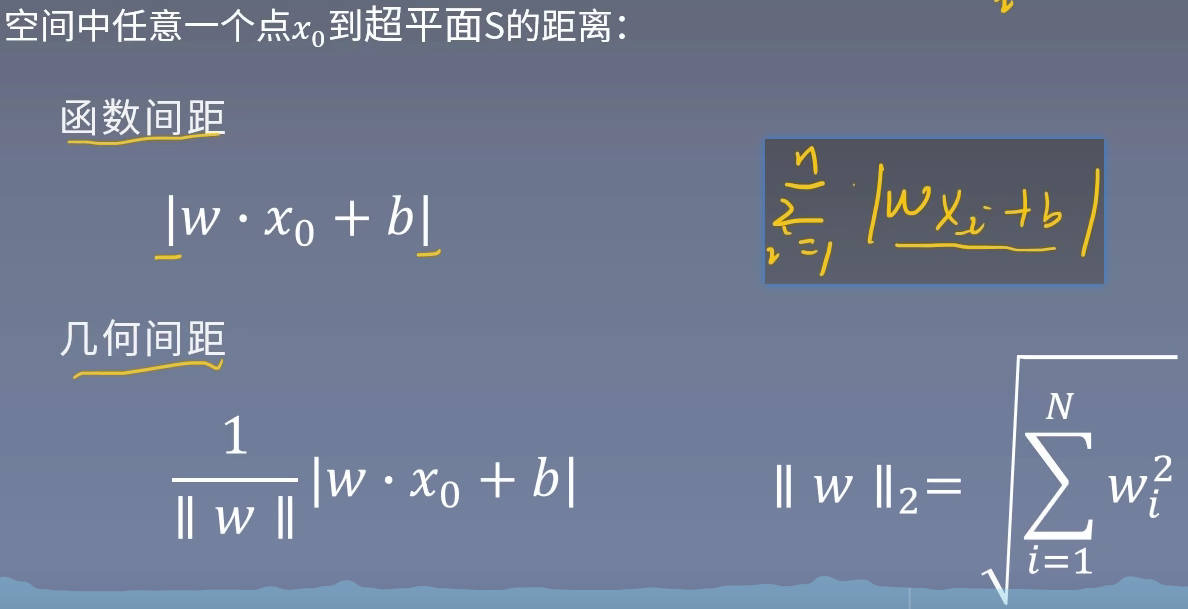## 函数间隔

$\sum_{i=1}^{N} \left | w^{T} x_{i} + b \right |$

## 使用几何间隔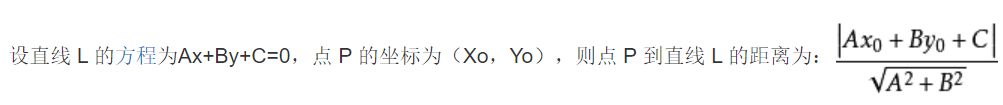$-y_{i} (w^{T}x+b )>=0$

$y_{i}$代表标签，正例为+1，反例为-1。$w^{T}x_{i} +b$代表预测数结果，若$w^{T}x_{i} +b>0$代表预测为正例，<0代表预测是负例。如果是错误数据，则上述的公式一定是>=0的。

### 为什么感知机不使用函数间隔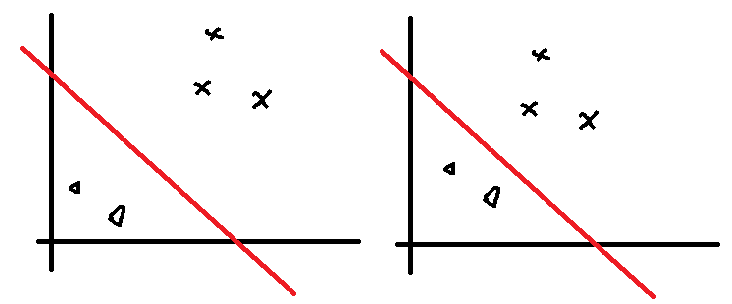$w^{T} x+b=1$

$\frac{1}{2}w^{T} x+\frac{1}{2}b=\frac{1}{2}$

$\frac{\left | 2w^{T} x+2b \right | }{\sqrt{\sum_{i=1}^{N}(2w^{T}_{i} )^{2} } } = \frac{2\left | w^{T}x+b \right | }{2\sqrt{\sum_{i=1}^{N}(w^{T})^{2} } }= \frac{\left | w^{T}x+b \right | }{\sqrt{\sum_{i=1}^{N}(w^{T})^{2} } }$

$-\frac{1}{\left | \left | w \right | \right | } y_{i} (w^{T}x+b )$

$-\frac{1}{\left | \left | w \right | \right | } \sum_{x_{i}\in M }^{} y_{i} (w^{T}x+b )$

## 感知机的损失函数

$L(w,b)=-\sum_{x_i\in M}y_i(w^Tx+b)$

# 感知机学习算法

## 感知机学习算法的原始形式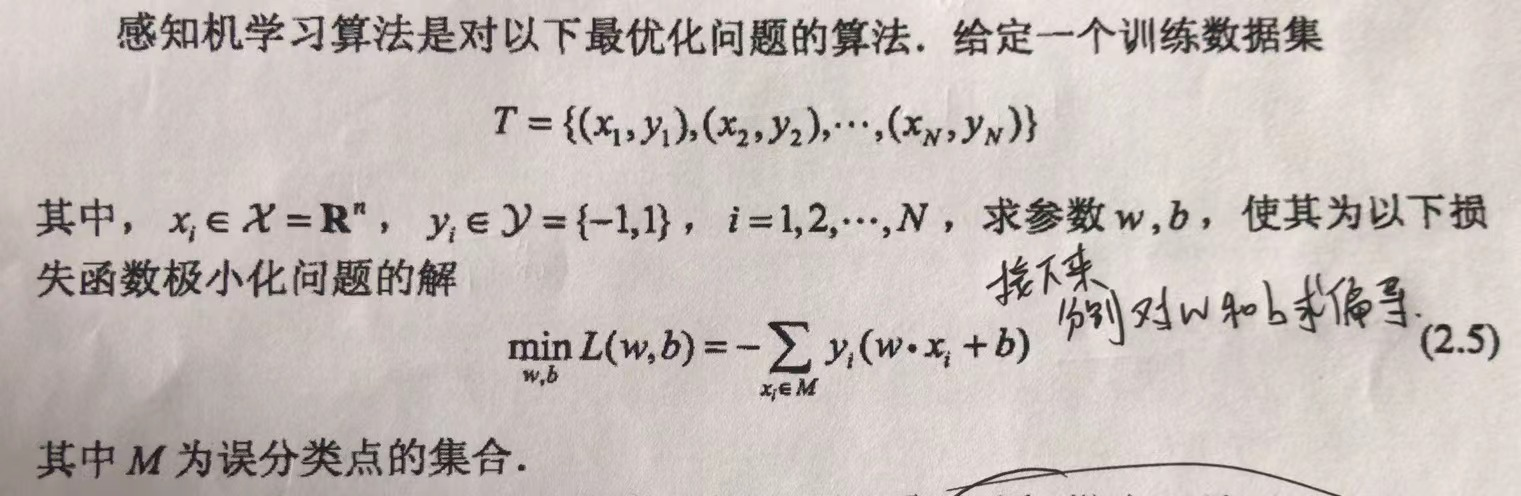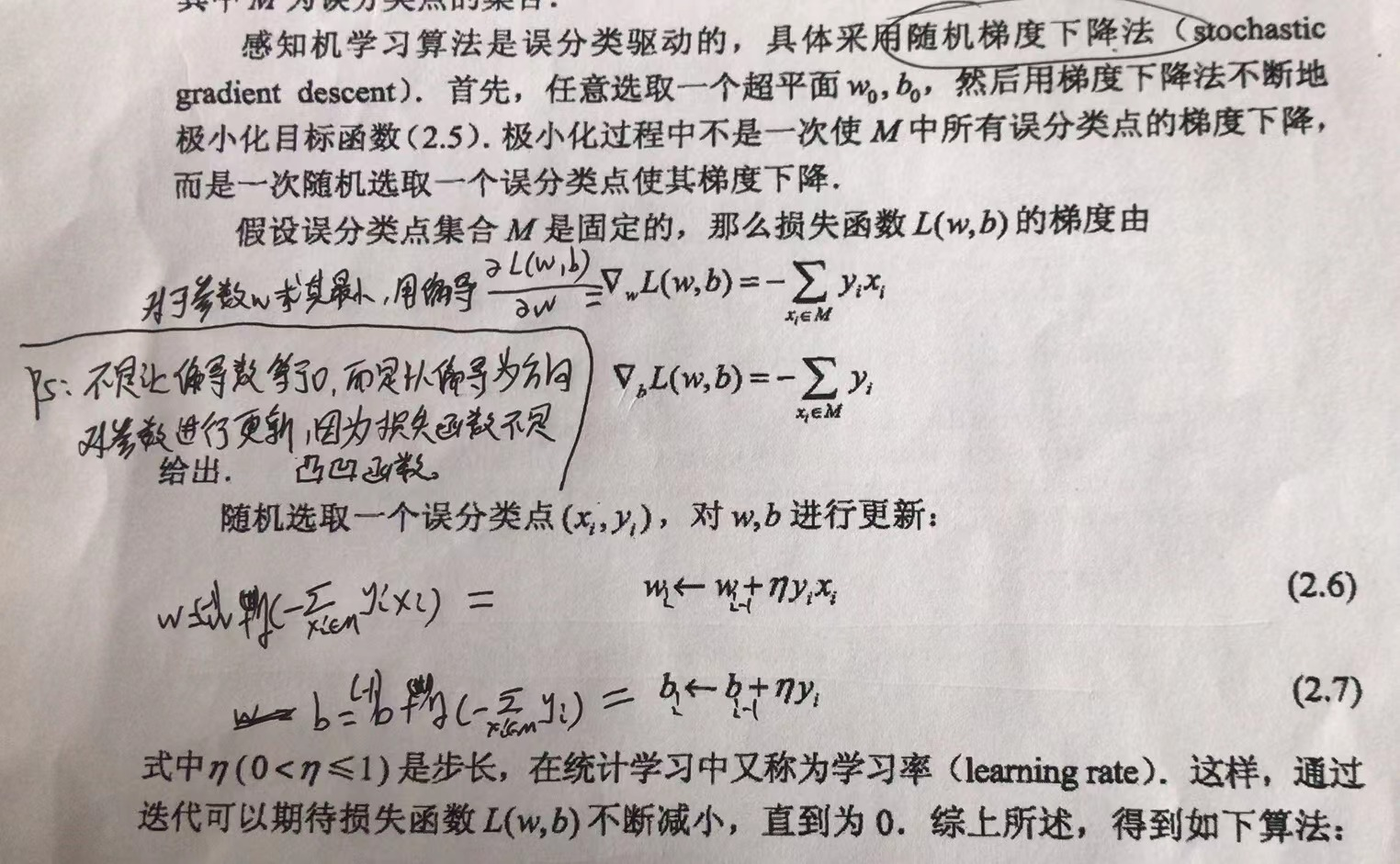• 随机任选一个超平面$w_0,b_0$，一般都初始化为0

• 在训练集中选取数据$(x_i,y_i)$

• 如果$y_i(w^Tx_i+b)\le 0$，则更新w和b：

$w_{i} =w_{i-1}+\eta y_ix_i$
$b_{i}=b_{i-1}+\eta y_i$

• 转至第二步，直到训练集中没有误分点

## 感知机学习算法的对偶形式（暂时跳过了）

$w=\sum_{i=1}^N\alpha_iy_ix_i\\b=\sum_{i=1}^N\alpha_iy_i$

• 令$\alpha=\boldsymbol 0, b=0$

• 在训练集中选取数据$(x_i,y_i)$

• 如果$y_i(\sum_{j=1}^N\alpha_jy_jx_jx_i+b)\le 0$，则更新w和b：

$\alpha=\alpha+\eta\\b=b+\eta y_i$

• 转至第二步，知道训练集中没有误分点

# 书中例子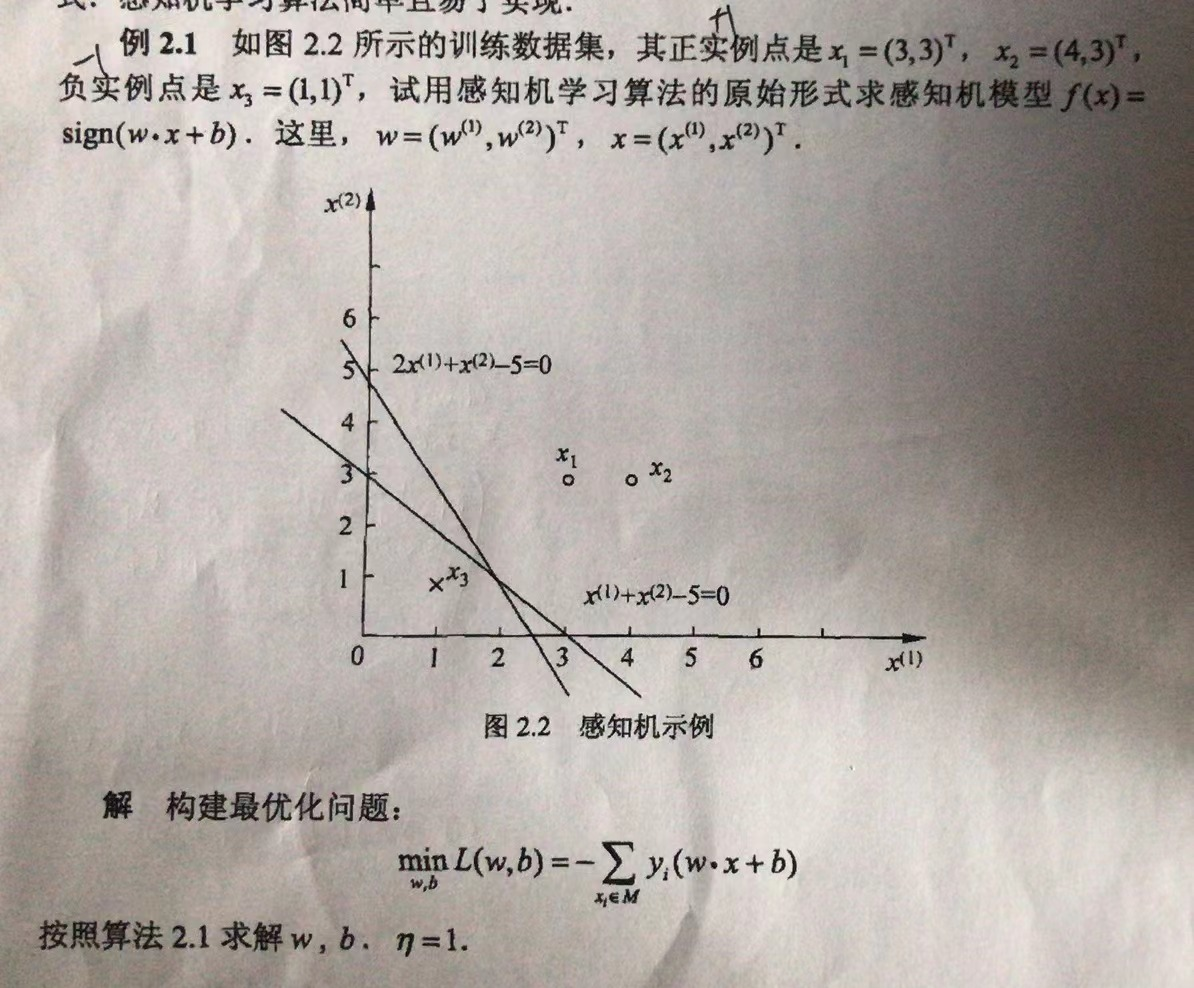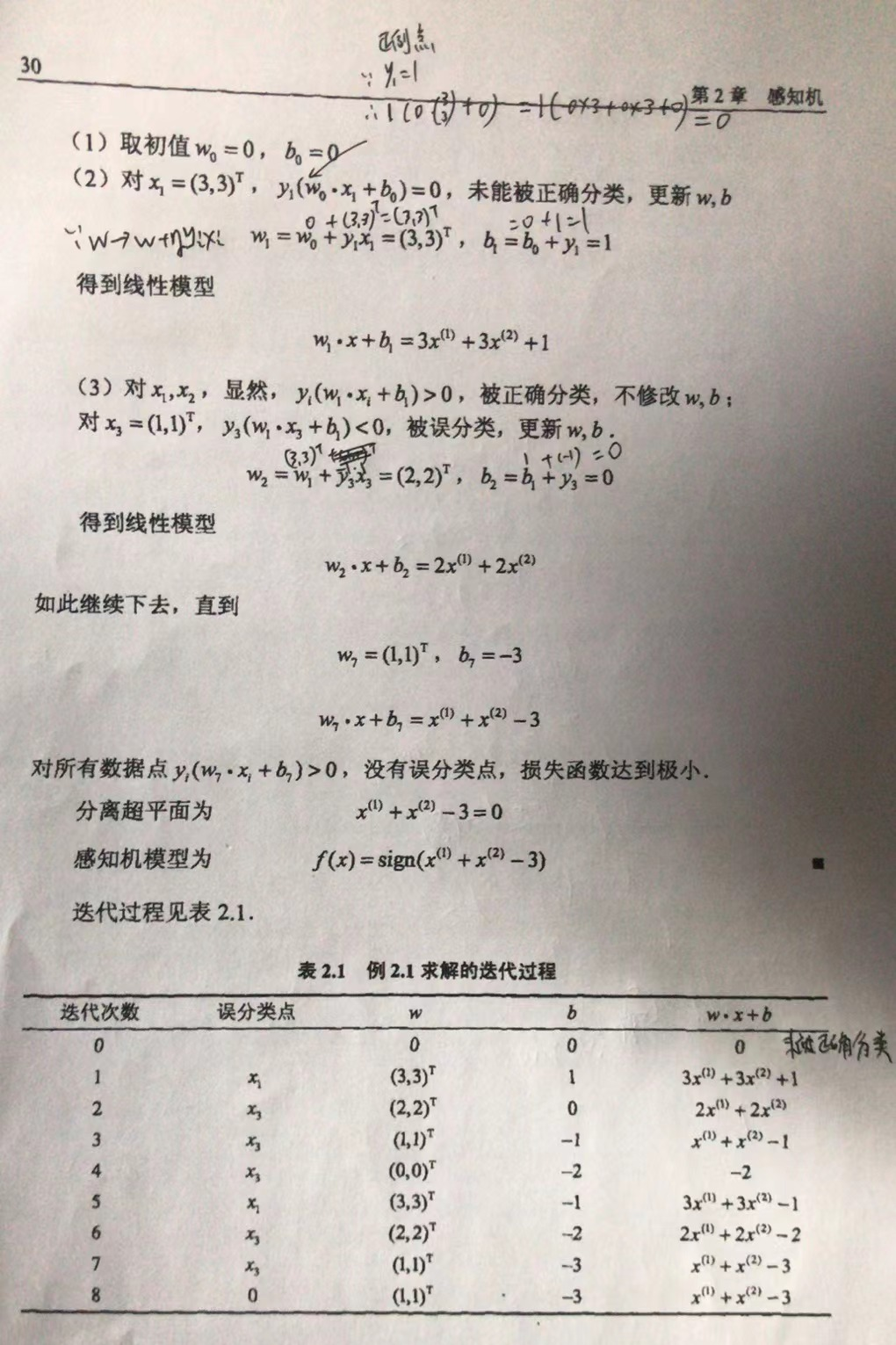## 编程解：

import numpy as np
import matplotlib.pyplot as plt
class Perception():
'''
感知机算法，包括原始形式和对偶形式
'''
def __init__(self):
self.learing_rate = 1
def train(self, data, labels):
'''
原始形式的感知机算法，输入数据必须线性可分
:param data: (n*m),n为样本个数，m为特征个数
:param labels: 样本标签
'''
data_size = data.shape
#初始化w,b
self.w =  * (data.shape)
self.b = 0
y_pred = np.sign((np.dot(data, self.w) + self.b))
#是否有误分类标记，当有误分类样本时执行以下循环
flag = (y_pred == labels).all()
while not flag:
for i in range(data_size):
y_pred = np.sign((np.dot(data, self.w) + self.b))
if y_pred[i]*labels[i] <= 0:
self.w += self.learing_rate * data[i] * labels[i]
self.b += self.learing_rate * labels[i]
print(self.w,self.b)
flag = (y_pred == labels).all()
def get_gram_matrix(self, data):
#计算格拉姆矩阵
data_size = data.shape
gram_matrix = np.zeros((data_size, data_size))
for i in range(data_size):
for j in range(data_size):
gram_matrix[i, j] = np.dot(data[i], data[j])
self.gram_matrix = gram_matrix
def dual_output(self, x_index, data_size, labels):
'''
计算第x_index个样本的对偶形式的输出，这里不进行符号函数运算
:param x_index: 样本索引
:param data_size: 样本个数
:param labels: 样本标签
'''
output = self.b
for i in range(data_size):
output += (self.alpha[i] * labels[i] * self.gram_matrix[i, x_index])
return output
def train_dual(self, data, labels):
#d对偶形式的感知机算法
self.b = 0
self.get_gram_matrix(data)
data_size = data.shape
self.alpha =  * data_size
flag = False
while not flag:
y_pred =  * data_size
for i in range(data_size):
y_pred[i] = np.sign(self.dual_output(i, data_size, labels))
if labels[i] * y_pred[i] <= 0:
self.alpha[i] += self.learing_rate
self.b += self.learing_rate * labels[i]
print(self.alpha,self.b)
flag = (y_pred == labels).all()
if __name__ == '__main__':
# data1中第一列表示x轴坐标；第二列代表y轴作表；第三列表示标签，1表示正例，-1代表反例。
data1 = np.array([[3, 3, 1], [4, 3, 1], [1, 1, -1]])    # 书中例题数据检验算法
# numpy切片：https://www.runoob.com/numpy/numpy-indexing-and-slicing.html
data = data1[..., 0:2]
labels = data1[..., -1]
model = Perception()
# 使用原始形式（还有一种对偶形式代码写了，但暂时不研究）
model.train(data, labels)
# 画图
# 轴范围 0~5
plt.xlim(0, 5)
plt.ylim(0, 5)
x = data1[..., 0]
y = data1[..., 1]
labl = data1[..., 2]
# 参考:https://www.codenong.com/12487060/
colors = ['red' if l == 1 else 'green' for l in labl]
plt.scatter(x, y, c=colors)
# python如何根据斜率和截距画直线？:https://www.3rxing.org/question/ca80d8505f642814980.html
print("model.w：", model.w)
print("model.w：", model.w)
print("model.b：", model.b)
k = - (model.w / model.w)
print("斜率k：", k)
b = -model.b / model.w
print("截距b：", b)
# 生成x的等差数列0-10之间取100个数
xx = np.linspace(0, 5, 100)
# 生成每个x对应的y
y = k * xx + b
# 画直线
plt.plot(xx, y)
plt.show()
# #随机生成数据,在y=x附近生成数据（暂时不做）
# x = np.linspace(0,50,50)
# y1 = x + np.random.randint(1,5,50)
# y2 = x + np.random.randint(-5,-1,50)
# data_positive = np.c_[x,y1]
# data_negative = np.c_[x,y2]
# labels_positive = np.ones(50)
# labels_negative = -np.ones(50)
# data = np.r_[data_positive,data_negative]
# labels = np.r_[labels_positive,labels_negative]
#
# model = Perception()
# model.train(data,labels)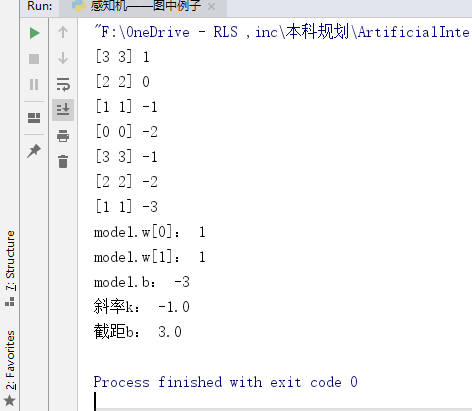《统计学习方法》李航

https://www.bilibili.com/video/BV1i4411G7Xv?p=2

https://github.com/1033020837/Basic4AI

https://ld246.com/article/1585574258471